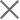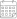Subscribe

### Indexes

May 2023 | Electricity Market Review03 July 2023

In May 2023, Georgian power plants generated 1,325 mln. kWh of electricity. This represents a 9% decrease in the total generation compared to the previous year (in May 2022, the total generation was 1,461 mln. kWh). The decrease in generation on a yearly basis comes from a fall of 9% in Hydro and 6% in wind power, while thermal power generation increased by 89%.

On a monthly basis, the generation increased by approximately 6% (in April 2023, the total generation was 1,249 mln. kWh) (Figure 1). The monthly rise in total generation is induced by a 15% increase in hydropower generation, while thermal and wind power generation decreased by 99% and 14%, respectively.

The consumption of electricity on the local market was 1,030 mln. kWh (-11% compared to May 2022, and -1% compared to April 2023) (Figure 1). In May 2023, power generation exceeded consumption by 295 mln. kWh which was 22% of the total generation and 29% of the total consumption (in May 2022, the difference between the total generation and the consumption resulted in a surplus of 307 mln. kWh, around 21% of the total generation and 27% of the total consumption for the month).